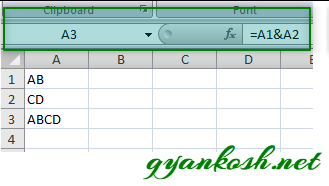PROVIDING ACCURATE, RELIABLE AND STRAIGHT TO THE POINT KNOWLEDGE ABOUT DIFFERENT IT TOOLS EXPLAINED WITH PRACTICAL EXAMPLES

# MANIPULATING TEXT IN EXCEL PART-I

## INTRODUCTION

Whenever we prepare any report in excel, we have two constituents in any report.

The Text portion and the Numerical portion.

But just storing the text and numbers doesn’t make the super reports. Many times we need to automate the process in the reports to minimize the effort and improve the accuracy.

Many functions are provided by the Excel which work on Text and give us the useful output as well. But few problems are still left on which we need to apply some tricks with the available tools.

We are going to learn many tricks and usage of function to perform different operations on the TEXT IN EXCEL.

In this article, we are going to learn how to combine text in excel.

Combining the text is required many times in the automation process of our reports.

## HOW TEXT IS HANDLED IN EXCEL?

TEXT is simply the group of characters and strings of characters which convey the information about the different data and numbers in Excel. Every character is connected with a code [ANSI].
Text comprises of the individual entity character which is the smallest bit which would be found in Excel.
We can perform the operations on the strings[Text] or the characters.
Characters are not limited to A to Z or a to z but many symbols are also included in this which we would see in the later part of the article.

TEXT IS AN INACTIVE NUMBER TYPE[FORMAT] IN EXCEL. ANYTHING STORED AS TEXT [NUMBER OR DATE] WON’T RESPOND TO ANY STANDARD FORMULAS OR FUNCTIONS BUT SPECIALLY DESIGNED TEXT FUNCTIONS. [EXCEPTIONS DO OCCUR IN CASE OF NUMBERS]
If we need to make anything inactive, such as Date to be non responding to the calculation, we put it as a text. Similarly if we want to avoid any calculations for a number it needs to be put as a text.

## COMBINE TEXT IN EXCEL

Combining text is excel is very useful technique.

Let us understand what we mean by combining the text.

The combining means to stick the text to one another. For example if one cell is having “ABCD” and the other is having the value as “EFGH”. If we combine them in the third cell the result will be “ABCDEFGH”.

The specific term used for sticking the text or combining the text or joining the text in the computer language is CONCATENATE which simply means to stick or append.

We can join the text in Excel in many ways.

2. #### USING CONCATENATE OR CONCAT FUNCTION

STEPS TO USE ” &” [CONCATENATION] OPERATOR TO JOIN TEXT IN EXCEL:
Just like the other operators in Excel such as + – / * : etc. we have “&” which is known as CONCATENATION OPERATOR.
We can make use of this operator directly to join any text in any number of cells.
For Example

Suppose we have a text ” AB” in cell A1 and “CD” in cell A2.
Let us join them and get the result in the cell A3 then we have to put the formula in A3 as
=A1 & A2
It’ll give us the result in A3 as ABCD.STEPS TO USE CONCATENATE OR CONCAT FUNCTION:
Just like the & operator , we have got two functions dedicated for this particular job of combining the text in Excel.
The functions are CONCAT or CONCATENATE.
CONCAT is just the advanced version of CONCATENATE FUNCTION, so we will cover both of them simultaneously.
• Select the cell where we want the result.
• Put the function as
• =Concatenate(cell 1, cell2, cell3,….) or =Concatenate(value1, value 2, value 3,…) .[ text value must be in “” to specify that it is text]
• The same can be done with the CONCAT FUNCTION.[This function is available in EXCEL 2019 onwards]
• Put the function as
• =Concat(cell 1, cell2, cell3,….) or =Concat(value1, value 2, value 3,…) .[ text value must be in “” to specify that it is text]

The difference between CONCAT FUNCTION and CONCATENATE FUNCTION is that CONCAT function can accept a complete Range of cells to act upon for example If we want to concatenate the cells from A1 to A5 we can simply write CONCAT(A1:A5).
EXAMPLE:
• We have the text A B C D E in the cells A1 B1 C1 D1 and E1 respectively.
• We would use CONCAT and CONCATENATE FUNCTION to combine these.
• We choose the cell D4 and D5 for the output.
• In D4 we put the formula as  =CONCATENATE(A1,B1,C1,D1,E1)
• The output is shown as ABCDE which was expected. We can use the CONCAT FORMULA for the same result.
• In D5 we use the formula as   =CONCAT(A1:E1) and the result is ABCDE as expected.

• ## WHAT IS EXCEL, HOW EXCEL WORKS, WRITE FORMULA IN EXCEL, EXAMPLE OF USING FORMULA IN EXCEL, ANIMATED EXAMLPE OF USING FORMULA IN EXCEL

LEARN

• EXCEL
• JAVASCRIPT
• MORE TO COME…

Encourage us by following at …

If you have any queries or suggestions or any problem which you would like us to solve for you, kindly mail at

[email protected]